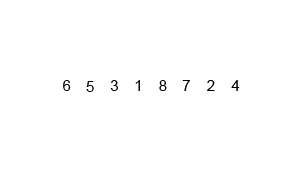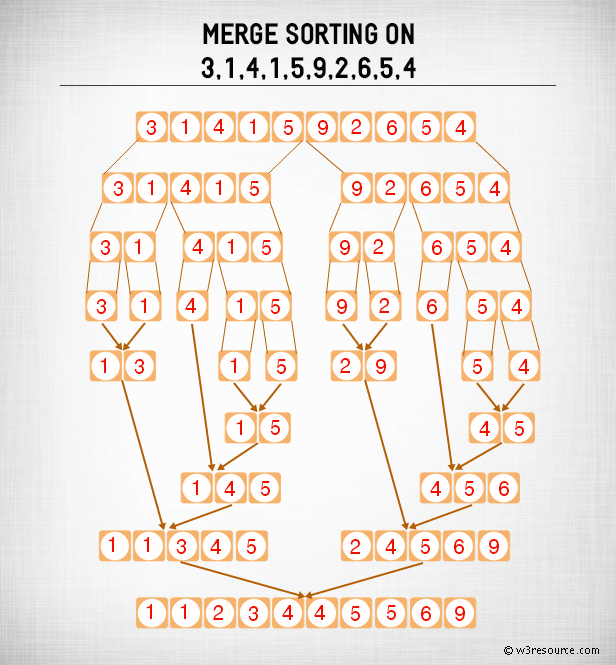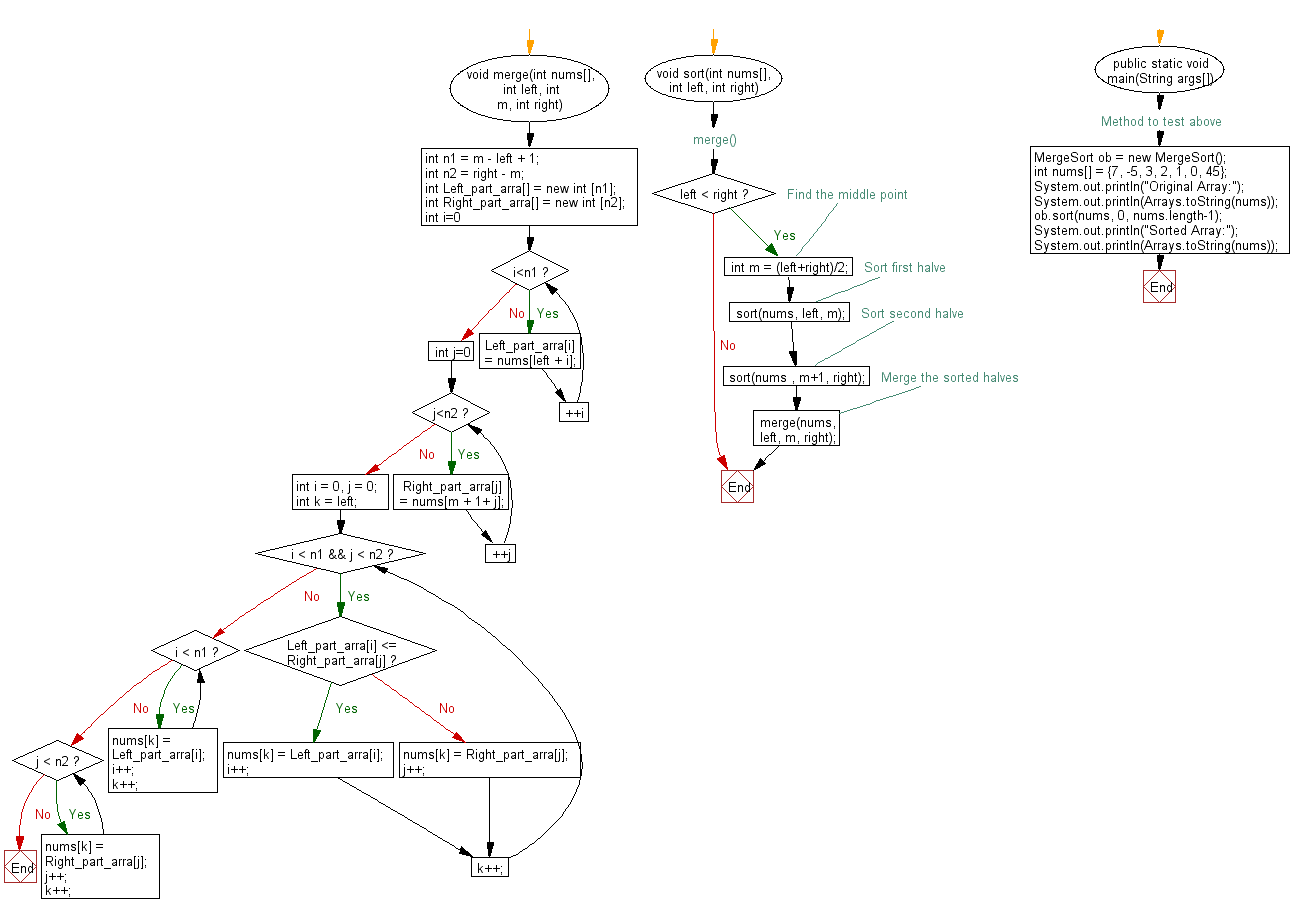﻿ Java exercises: Merge sort Algorithm. - w3resource# Java Exercises: Merge sort Algorithm

## Java Sorting Algorithm: Exercise-4 with Solution

Write a Java program to sort an array of given integers using Merge sort Algorithm.

According to Wikipedia "Merge sort (also commonly spelled mergesort) is an O (n log n) comparison-based sorting algorithm. Most implementations produce a stable sort, which means that the implementation preserves the input order of equal elements in the sorted output."

Algorithm:

Conceptually, a merge sort works as follows :

• Divide the unsorted list into n sublists, each containing 1 element (a list of 1 element is considered sorted).
• Repeatedly merge sublists to produce new sorted sublists until there is only 1 sublist remaining. This will be the sorted list.

An example of merge sort:Merge Sort: Pictorial PresentationSample Solution:

Java Code:

``````import java.util.Arrays;
class MergeSort
{
void merge(int nums[], int left, int m, int right)
{
int n1 = m - left + 1;
int n2 = right - m;

int Left_part_arra[] = new int [n1];
int Right_part_arra[] = new int [n2];

for (int i=0; i<n1; ++i)
Left_part_arra[i] = nums[left + i];
for (int j=0; j<n2; ++j)
Right_part_arra[j] = nums[m + 1+ j];

int i = 0, j = 0;

int k = left;
while (i < n1 && j < n2)
{
if (Left_part_arra[i] <= Right_part_arra[j])
{
nums[k] = Left_part_arra[i];
i++;
}
else
{
nums[k] = Right_part_arra[j];
j++;
}
k++;
}

while (i < n1)
{
nums[k] = Left_part_arra[i];
i++;
k++;
}

while (j < n2)
{
nums[k] = Right_part_arra[j];
j++;
k++;
}
}

// merge()
void sort(int nums[], int left, int right)
{
if (left < right)
{
// Find the middle point
int m = (left+right)/2;

// Sort first halve
sort(nums, left, m);
// Sort second halve
sort(nums , m+1, right);

// Merge the sorted halves
merge(nums, left, m, right);
}
}

// Method to test above
public static void main(String args[])
{
MergeSort ob = new MergeSort();
int nums[] = {7, -5, 3, 2, 1, 0, 45};
System.out.println("Original Array:");
System.out.println(Arrays.toString(nums));
ob.sort(nums, 0, nums.length-1);
System.out.println("Sorted Array:");
System.out.println(Arrays.toString(nums));
}

}
```
```

Sample Output:

```Original Array:
[7, -5, 3, 2, 1, 0, 45]
Sorted Array:
[-5, 0, 1, 2, 3, 7, 45]
```

Flowchart:Java Code Editor:

What is the difficulty level of this exercise?

﻿

## Java: Tips of the Day

Array vs ArrayLists:

The main difference between these two is that an Array is of fixed size so once you have created an Array you cannot change it but the ArrayList is not of fixed size. You can create instances of ArrayLists without specifying its size. So if you create such instances of an ArrayList without specifying its size Java will create an instance of an ArrayList of default size.

Once an ArrayList is full it re-sizes itself. In fact, an ArrayList is internally supported by an array. So when an ArrayList is resized it will slow down its performance a bit as the contents of the old Array must be copied to a new Array.

At the same time, it's compulsory to specify the size of an Array directly or indirectly while creating it. And also Arrays can store both primitives and objects while ArrayLists only can store objects.

Ref: https://bit.ly/3o8L2KH